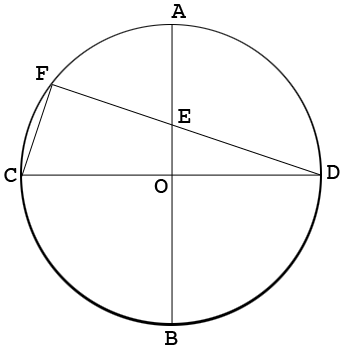SEARCH HOMEMath Central Quandaries & QueriesQuestion from Ahamed: the diameters of a circle AB and CD intersect each other at O perpendicularly. Then a chord DF is drawn. AB and DF intersect at E. DE=6cn and EF=2cm.find the area of the circleHi Ahamed,

Here is my diagram.Since the two diagonals intersect at the center of the circle $O$ must be the center of the circle.

What do you know about the angle $DFC?$

What do you know about the triangles $DFC$ and $DEO?$

Penny* Registered trade mark of Imperial Oil Limited. Used under license.Math Central is supported by the University of Regina and the Imperial Oil Foundation.